# Angular Momentum of a particle The angular momentum

• Slides: 24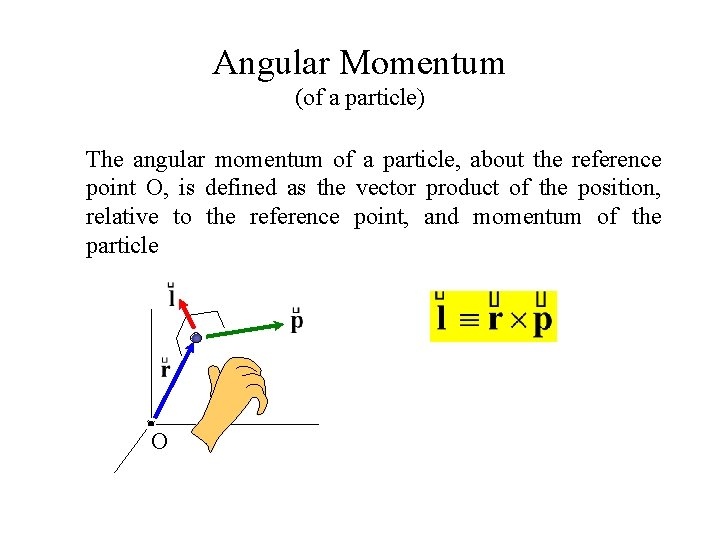Angular Momentum (of a particle) The angular momentum of a particle, about the reference point O, is defined as the vector product of the position, relative to the reference point, and momentum of the particle O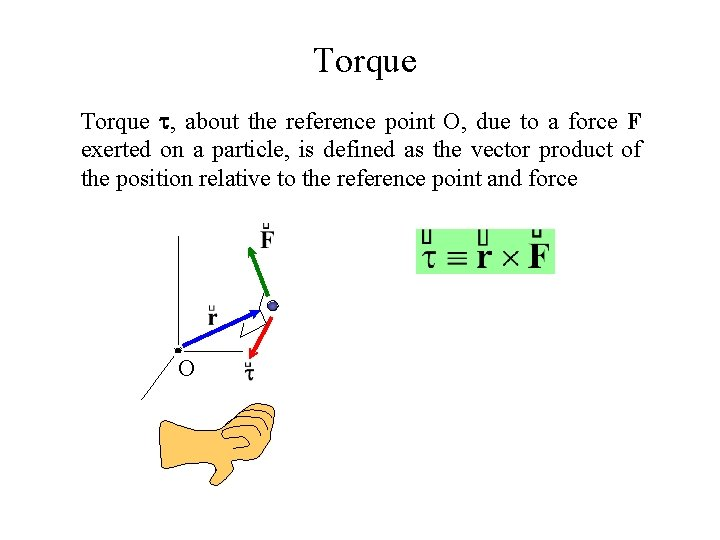Torque , about the reference point O, due to a force F exerted on a particle, is defined as the vector product of the position relative to the reference point and force O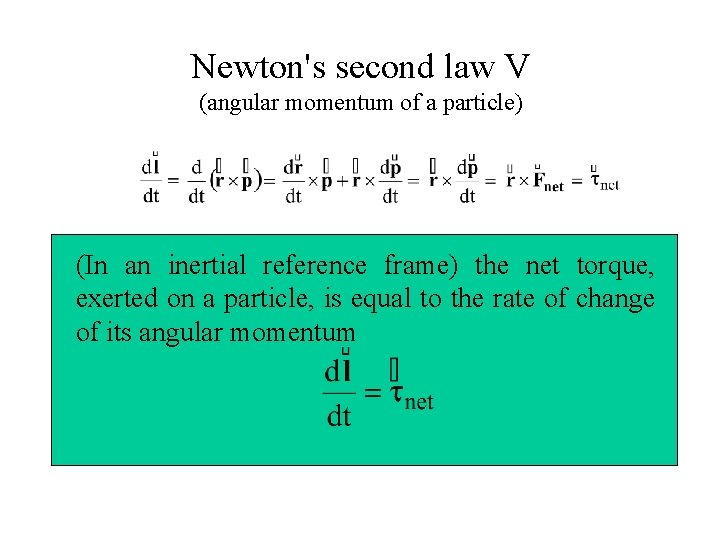Newton's second law V (angular momentum of a particle) (In an inertial reference frame) the net torque, exerted on a particle, is equal to the rate of change of its angular momentumExample. Kepler’s second law d. A The gravitational torque (about the sun) exerted by the sun on the planets is a zero vector. LNewton's second law VI (angular momentum of a system) (In an inertial reference frame) the net external torque, exerted on a system of particles, is equal to the rate of change of its (total) angular momentum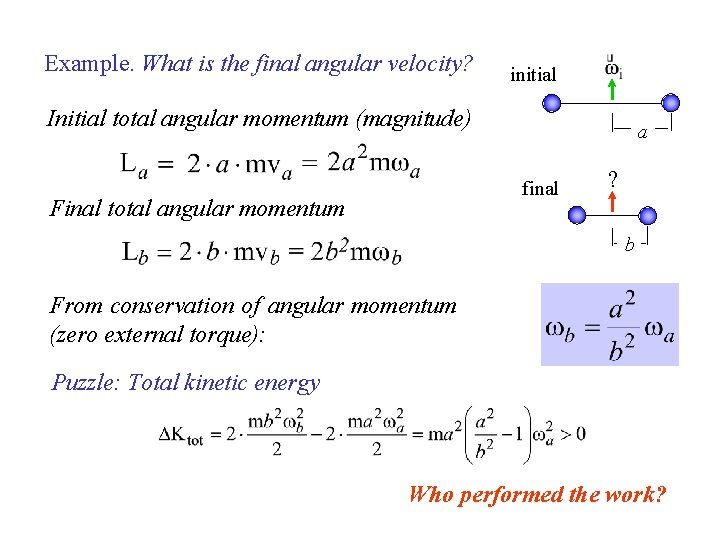Example. What is the final angular velocity? initial Initial total angular momentum (magnitude) a final Final total angular momentum ? b From conservation of angular momentum (zero external torque): Puzzle: Total kinetic energy Who performed the work?Rigid Body A system in which the relative position of all particles is time independent is called a rigid body. The motion can be considered as a superposition of the translational motion of a point and the rotational motion around the point. A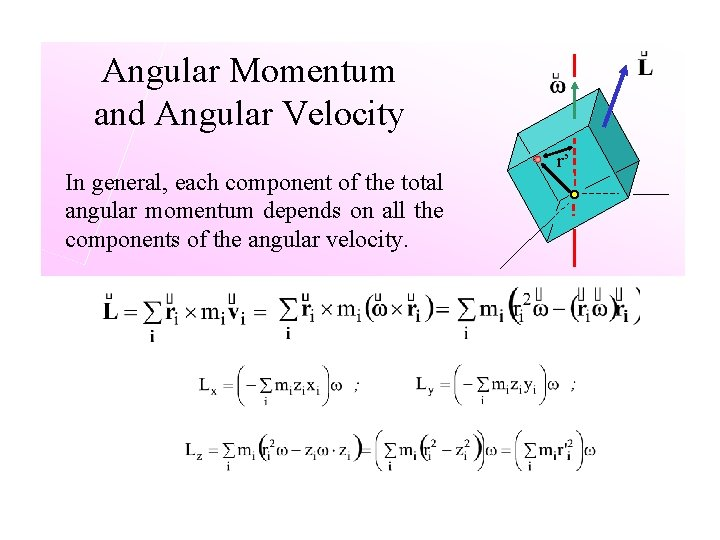Angular Momentum and Angular Velocity In general, each component of the total angular momentum depends on all the components of the angular velocity. r’effect of symmetry Only for object with appropriate symmetry the direction of angular momentum is consistent with the direction of angular velocity of the object is called the moment of inertia (rotational inertia) of the body about the axis of rotation.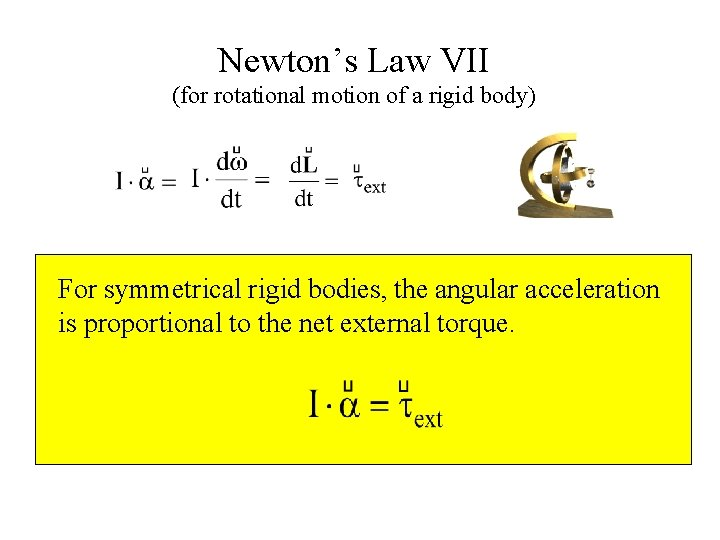Newton’s Law VII (for rotational motion of a rigid body) For symmetrical rigid bodies, the angular acceleration is proportional to the net external torque.Fixed and Instantaneous Axis of Rotation (Newton’s second law VIII) The angular acceleration, of an object rotating about a fixed axis or instantaneous, is proportional to the component, along the axis of rotation, of the net external torqueMoment of Inertia (rotational inertia) A system of particles: r’ ri’ dm m i continuous body A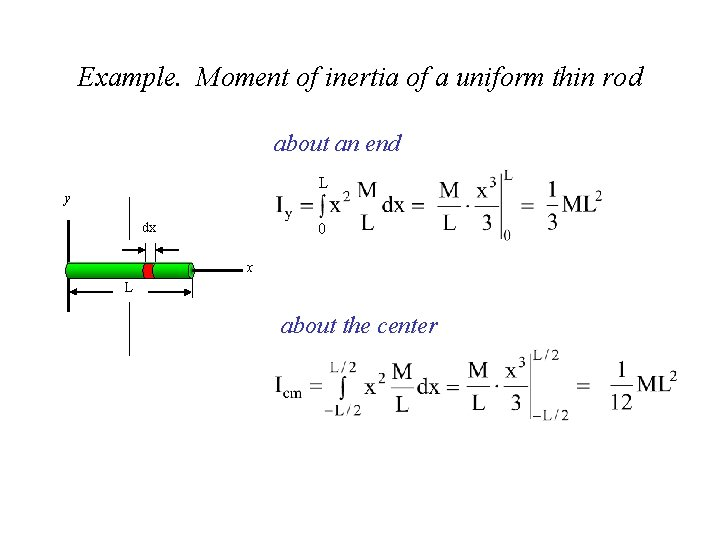Example. Moment of inertia of a uniform thin rod about an end L y 0 dx x L about the center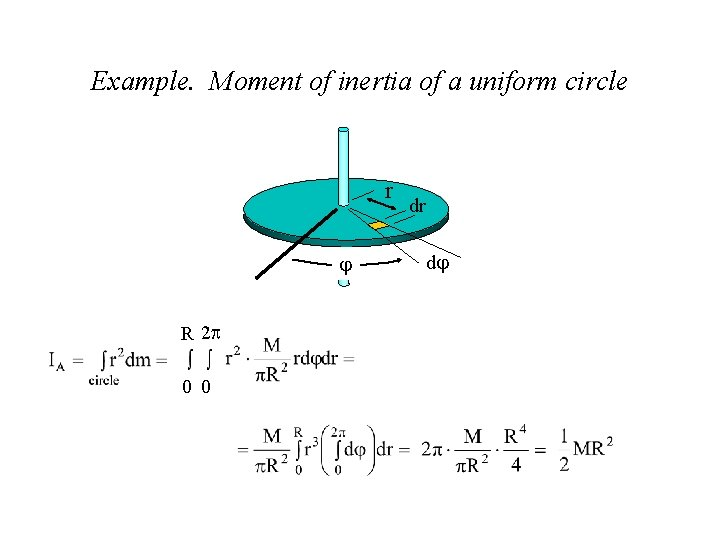Example. Moment of inertia of a uniform circle r R 2 0 0 dr d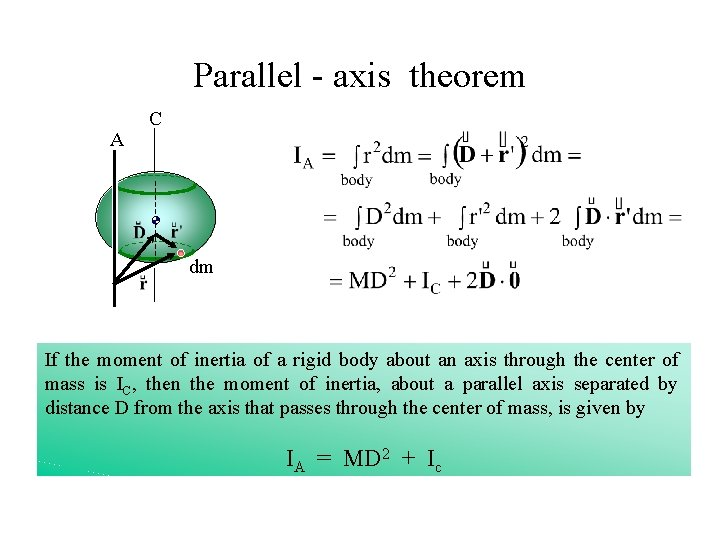Parallel - axis theorem A C dm If the moment of inertia of a rigid body about an axis through the center of mass is IC, then the moment of inertia, about a parallel axis separated by distance D from the axis that passes through the center of mass, is given by IA = MD 2 + Ic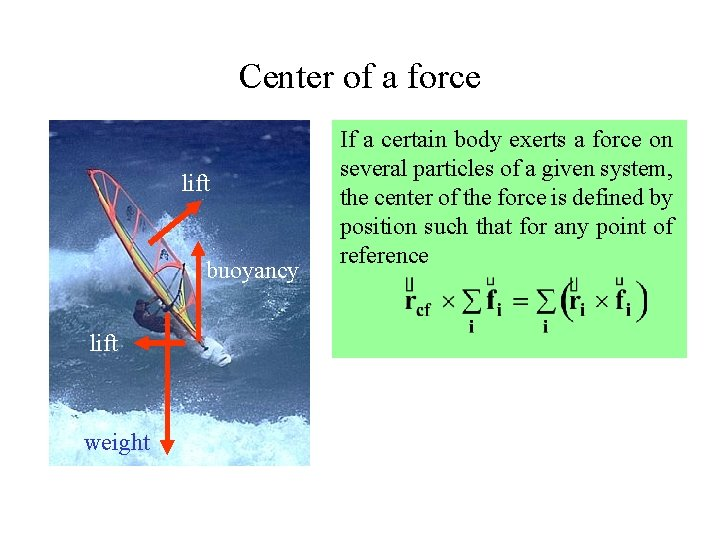Center of a force lift buoyancy lift weight If a certain body exerts a force on several particles of a given system, the center of the force is defined by position such that for any point of reference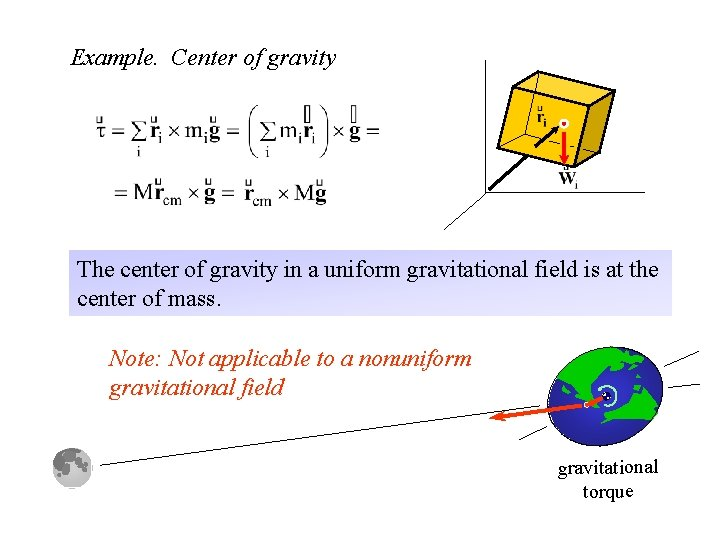Example. Center of gravity The center of gravity in a uniform gravitational field is at the center of mass. Note: Not applicable to a nonuniform gravitational field gravitational torque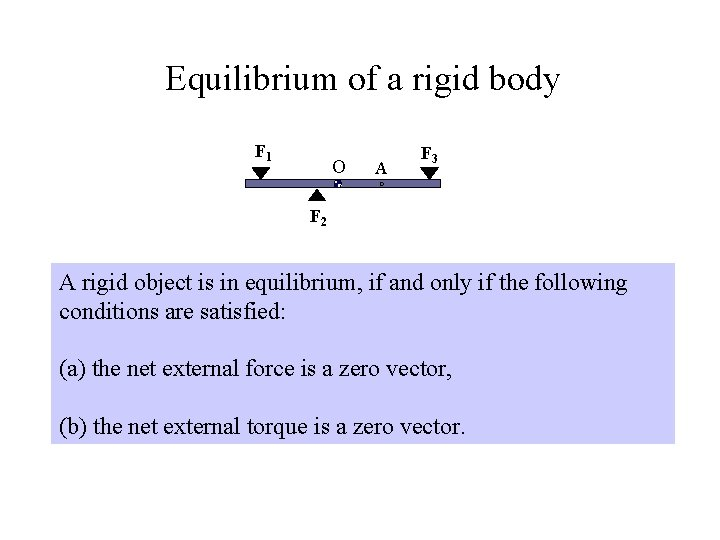Equilibrium of a rigid body F 1 O A F 3 F 2 A rigid object is in equilibrium, if and only if the following conditions are satisfied: (a) the net external force is a zero vector, (b) the net external torque is a zero vector.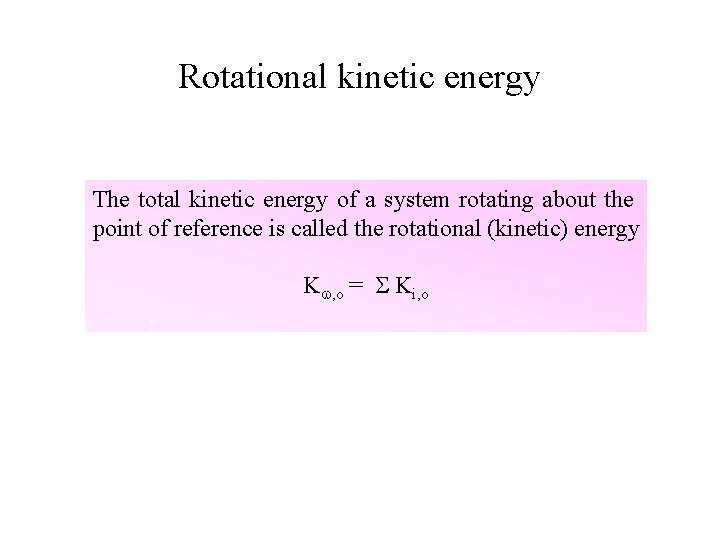Rotational kinetic energy The total kinetic energy of a system rotating about the point of reference is called the rotational (kinetic) energy K , o = Ki, orotational energy and angular velocity The rotational kinetic energy is related to the magnitude of angular velocity and the moment of inertia of the body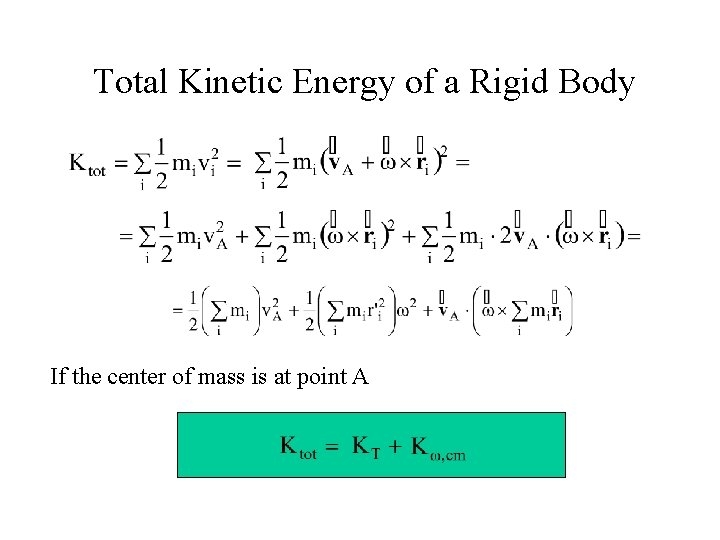Total Kinetic Energy of a Rigid Body If the center of mass is at point A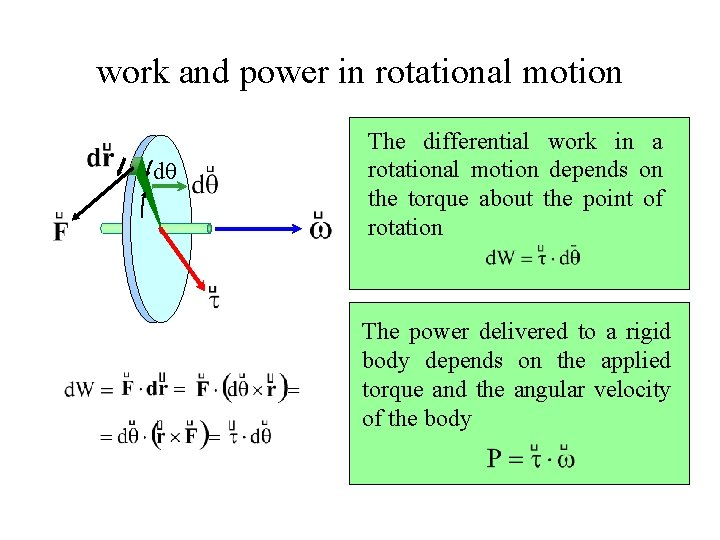work and power in rotational motion d The differential work in a rotational motion depends on the torque about the point of rotation The power delivered to a rigid body depends on the applied torque and the angular velocity of the bodyTransformation of torque A B F conclusion (total force) d If the total force applied to a body is zero, the torque of this force about any point has the same value. -F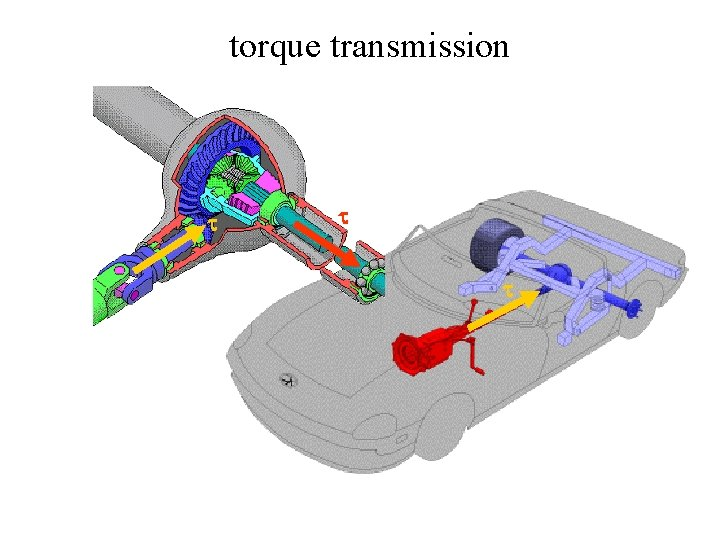torque transmission F